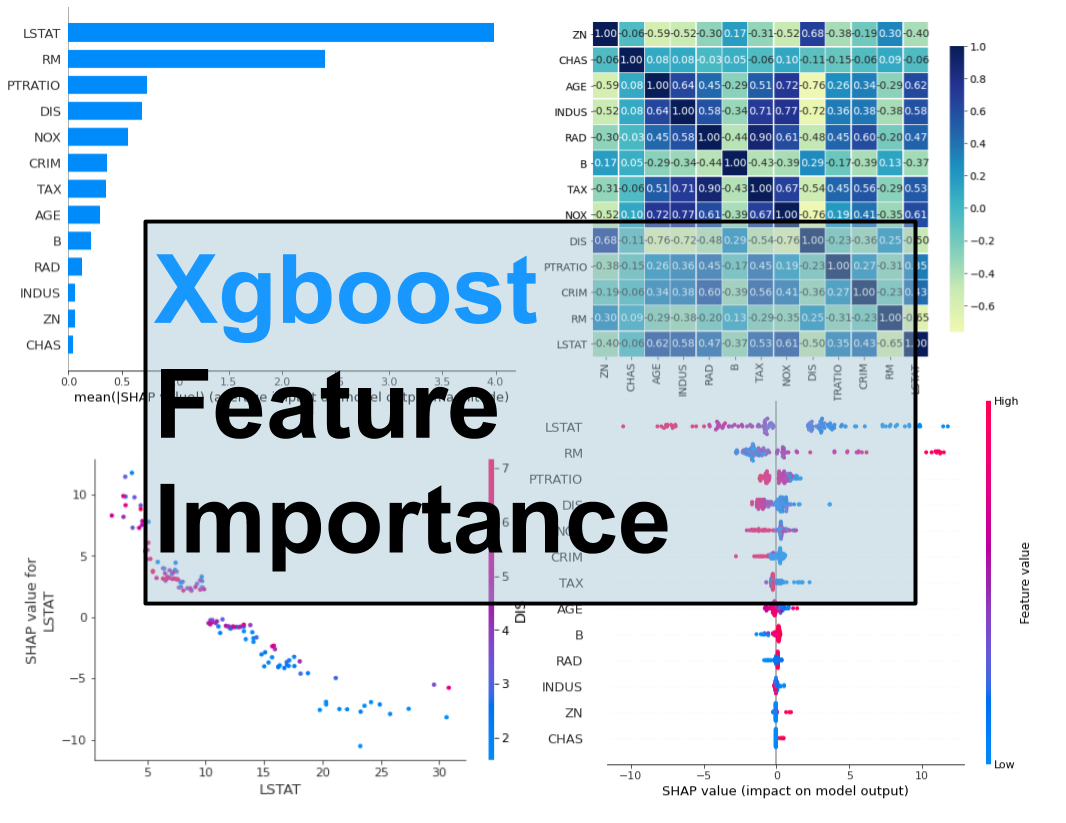Xgboost is a gradient boosting library. It provides parallel boosting trees algorithm that can solve Machine Learning tasks. It is available in many languages, like: C++, Java, Python, R, Julia, Scala. In this post, I will show you how to get feature importance from Xgboost model in Python. In this example, I will use `boston` dataset availabe in `scikit-learn` pacakge (a regression task).

You will learn how to compute and plot:

• Feature Importance built-in the Xgboost algorithm,
• Feature Importance computed with Permutation method,
• Feature Importance computed with `SHAP` values.

All the code is available as Google Colab Notebook. Happy coding!

## Xgboost Built-in Feature Importance

Let’s start with importing packages. Please note that if you miss some package you can install it with pip (for example, `pip install shap`).

``````import numpy as np
import pandas as pd
import shap

from sklearn.model_selection import train_test_split
from sklearn.inspection import permutation_importance
from matplotlib import pyplot as plt
import seaborn as sns # for correlation heatmap

from xgboost import XGBRegressor
``````

Load the `boston` data set and split it into training and testing subsets. The 75% of data will be used for training and the rest for testing (will be needed in permutation-based method).

``````boston = load_boston()
X = pd.DataFrame(boston.data, columns=boston.feature_names)
y = boston.target
X_train, X_test, y_train, y_test = train_test_split(X, y, test_size=0.25, random_state=12)
``````

Fitting the Xgboost Regressor is simple and take 2 lines (amazing package, I love it!):

``````xgb = XGBRegressor(n_estimators=100)
xgb.fit(X_train, y_train)
``````

I’ve used default hyperparameters in the Xgboost and just set the number of trees in the model (`n_estimators=100`).

To get the feature importances from the Xgboost model we can just use the `feature_importances_` attribute:

``````xgb.feature_importances_
``````
``````array([0.01690426, 0.00777439, 0.0084541 , 0.04072201, 0.04373369,
0.20451033, 0.01512331, 0.04763542, 0.01018296, 0.02332482,
0.04085794, 0.01299683, 0.52778   ], dtype=float32)
``````

It’s is important to notice, that it is the same API interface like for ‘scikit-learn’ models, for example in Random Forest we would do the same to get importances.

Let’s visualize the importances (chart will be easier to interpret than values).

``````plt.barh(boston.feature_names, xgb.feature_importances_)
``````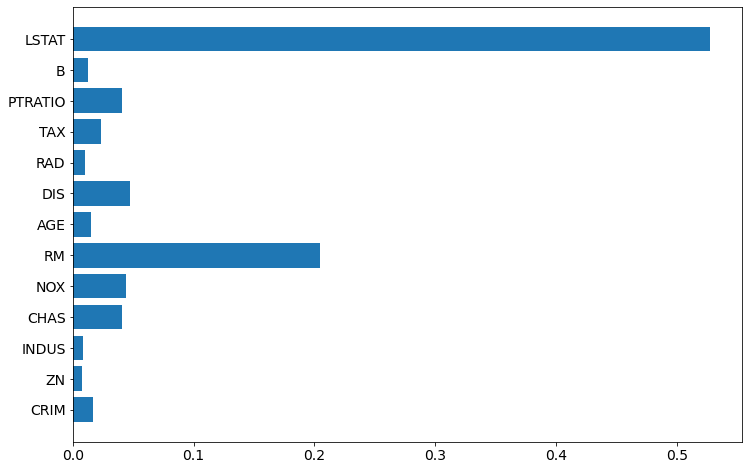To have even better plot, let’s sort the features based on importance value:

``````sorted_idx = xgb.feature_importances_.argsort()
plt.barh(boston.feature_names[sorted_idx], xgb.feature_importances_[sorted_idx])
plt.xlabel("Xgboost Feature Importance")
``````### About Xgboost Built-in Feature Importance

• There are several types of importance in the Xgboost - it can be computed in several different ways. The default type is `gain` if you construct model with `scikit-learn` like API (docs). When you access `Booster` object and get the importance with `get_score` method, then default is `weight`. You can check the type of the importance with `xgb.importance_type`.
• The `gain` type shows the average gain across all splits where feature was used.
• The `weight` shows the number of times the feature is used to split data. This type of feature importance can favourize numerical and high cardinality features. Be careful!
• There are also `cover`, `total_gain`, `total_cover` types of importance.

## Permutation Based Feature Importance (with `scikit-learn`)

Yes, you can use `permutation_importance` from `scikit-learn` on Xgboost! (`scikit-learn` is amazing!) It is possible because Xgboost implements the `scikit-learn` interface API. It is available in `scikit-learn` from version 0.22.

This permutation method will randomly shuffle each feature and compute the change in the model’s performance. The features which impact the performance the most are the most important one.

The permutation importance for Xgboost model can be easily computed:

``````perm_importance = permutation_importance(xgb, X_test, y_test)
``````

The visualization of the importance:

``````sorted_idx = perm_importance.importances_mean.argsort()
plt.barh(boston.feature_names[sorted_idx], perm_importance.importances_mean[sorted_idx])
plt.xlabel("Permutation Importance")
``````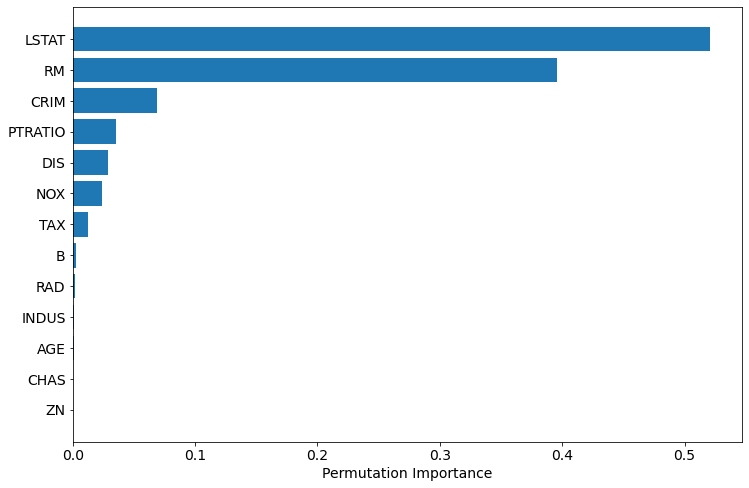The permutation based importance is computationally expensive (for each feature there are several repeast of shuffling). The permutation based method can have problem with highly-correlated features. Let’s check the correlation in our dataset:

``````def correlation_heatmap(train):
correlations = train.corr()

fig, ax = plt.subplots(figsize=(10,10))
sns.heatmap(correlations, vmax=1.0, center=0, fmt='.2f', cmap="YlGnBu",
square=True, linewidths=.5, annot=True, cbar_kws={"shrink": .70}
)
plt.show();

correlation_heatmap(X_train[boston.feature_names[sorted_idx]])
``````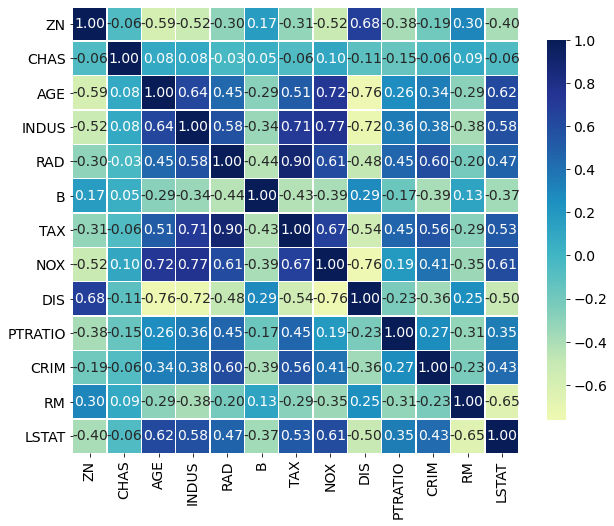Based on above results, I would say that it is safe to remove: `ZN`, `CHAS`, `AGE`, `INDUS`. Their importance based on permutation is very low and they are not highly correlated with other features (`abs(corr) < 0.8`).

In AutoML package `mljar-supervised`, I do one trick for feature selection: I insert random feature to the training data and check which features have smaller importance than a random feature. I remove those from further training. The trick is very similar to one used in the Boruta algorihtm.

## Feature Importance Computed with SHAP Values

The third method to compute feature importance in Xgboost is to use SHAP package. It is model-agnostic and using the Shapley values from game theory to estimate the how does each feature contribute to the prediction.

``````explainer = shap.TreeExplainer(xgb)
shap_values = explainer.shap_values(X_test)
``````

To visualize the feature importance we need to use `summary_plot` method:

``````shap.summary_plot(shap_values, X_test, plot_type="bar")
``````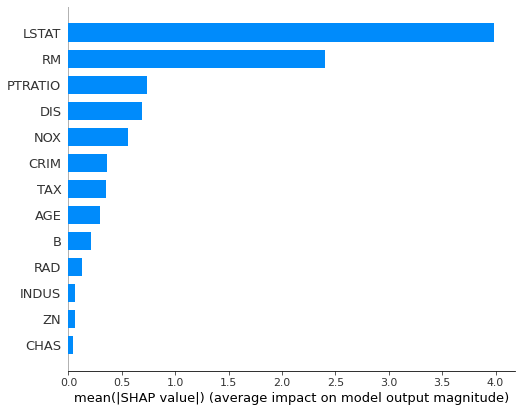The nice thing about `SHAP` package is that it can be used to plot more interpretation plots:

``````shap.summary_plot(shap_values, X_test)
``````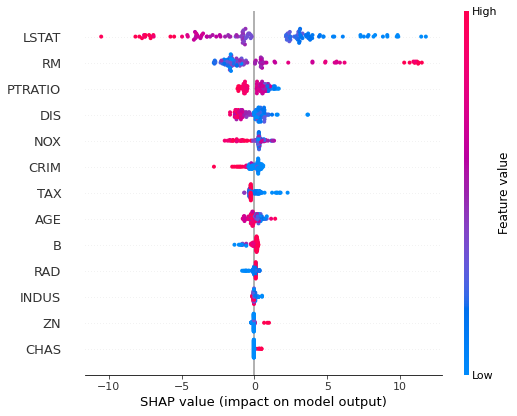``````shap.dependence_plot("LSTAT", shap_values, X_test)
``````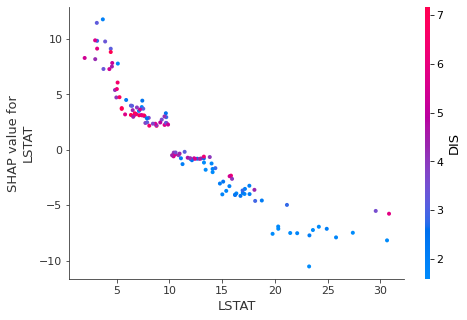The computing feature importances with SHAP can be computationally expensive.

## Summary

The are 3 ways to compute the feature importance for the Xgboost:

• built-in feature importance
• permutation based importance
• importance computed with SHAP values

In my opinion, it is always good to check all methods and compare the results. It is important to check if there are highly correlated features in the dataset. They can break the whole analysis.

## Important Notes

• The more accurate model is, the more trustworthy computed importances are.
• Feature importance is an approximation of how important features are in the data.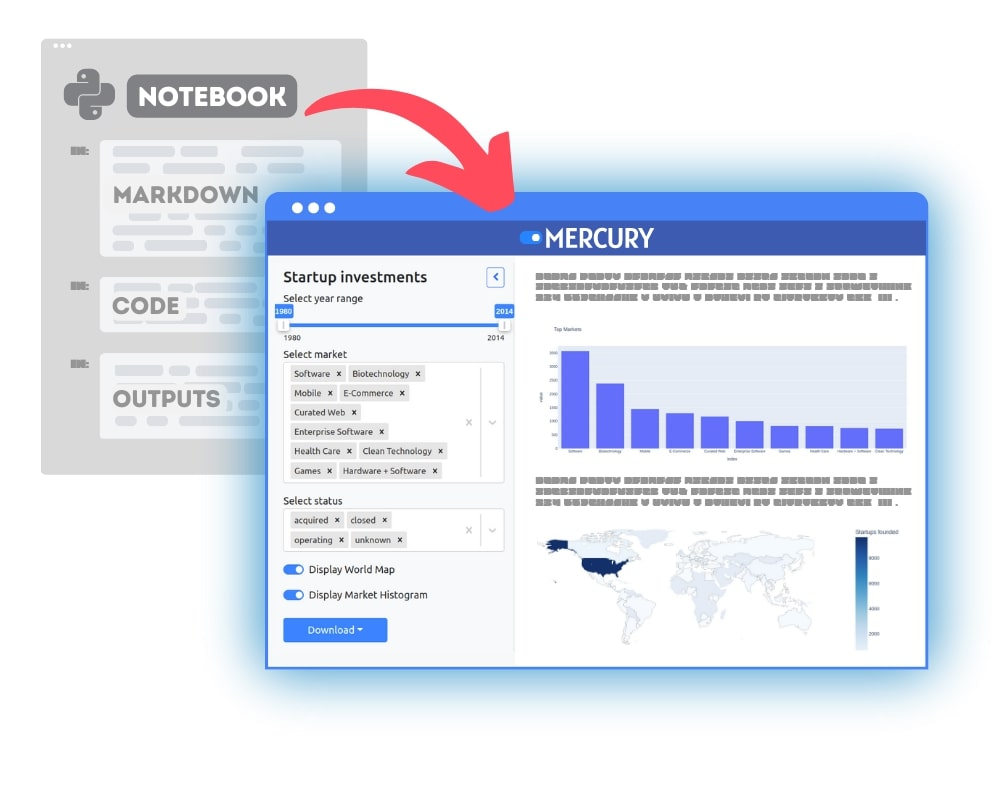### Convert Python Notebooks to Web Apps

We are working on open-source framework Mercury for converting Jupyter Notebooks to interactive Web Applications.### Articles you might find interesing